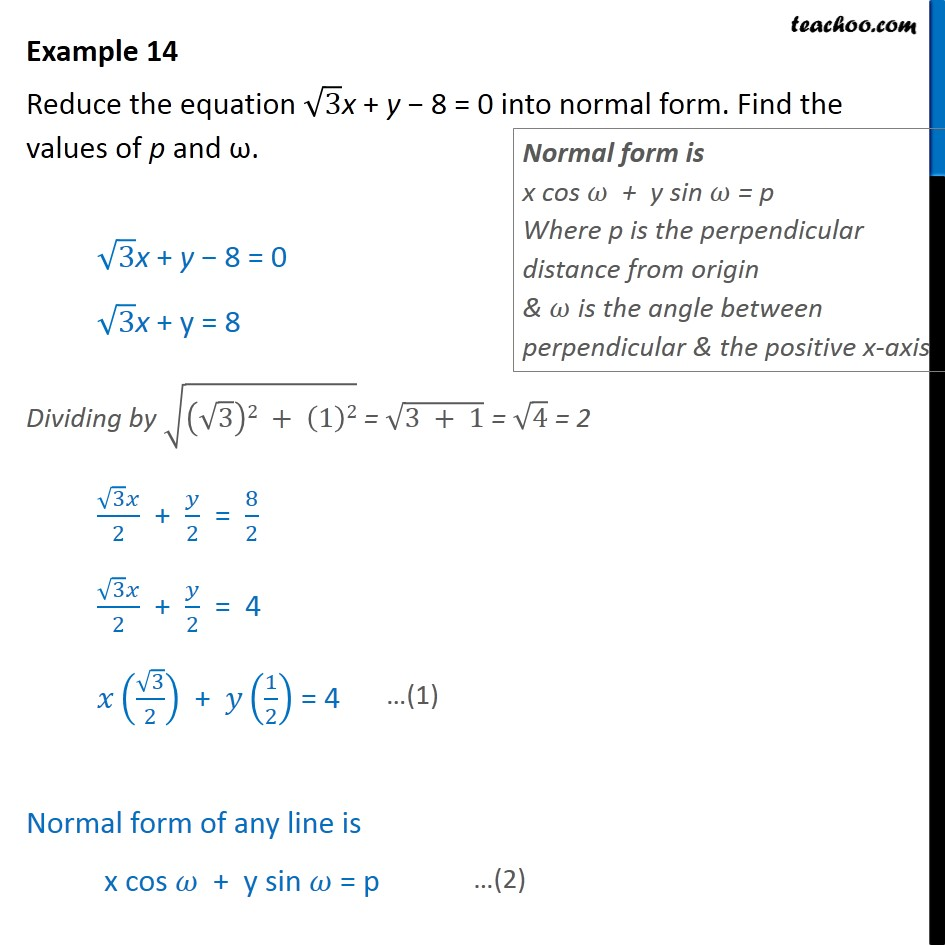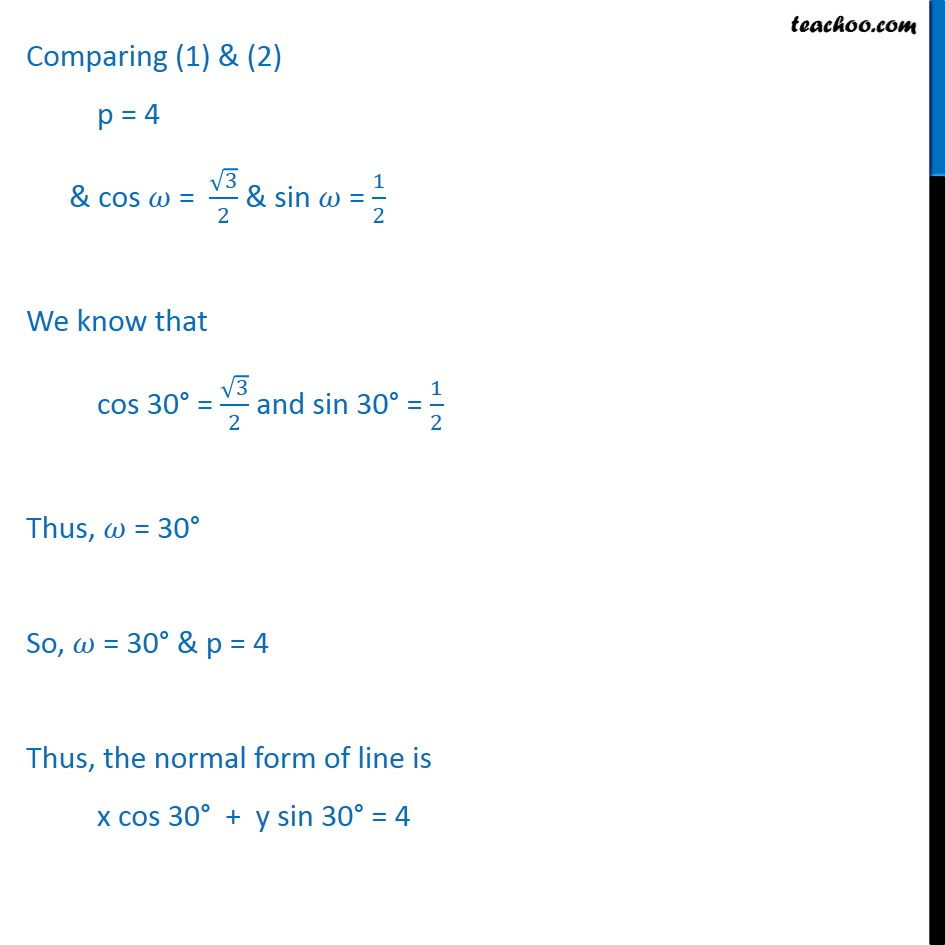1. Chapter 10 Class 11 Straight Lines
2. Serial order wise
3. Examples

Transcript

Example 14 Reduce the equation 3x + y 8 = 0 into normal form. Find the values of p and . 3x + y 8 = 0 " " 3 "x + y" = 8 Dividing by (( 3)2 + (1)2) = (3 + 1) = 4 = 2 ( 3 )/2 + /2 = 8/2 ( 3 )/2 + /2 = 4 ( 3/2) + (1/2) = 4 Normal form of any line is x cos + y sin = p Comparing (1) & (2) p = 4 & cos = 3/2 & sin = 1/2 We know that cos 30 = 3/2 and sin 30 = 1/2 Thus, = 30 So, = 30 & p = 4 Thus, the normal form of line is x cos 30 + y sin 30 = 4

Examples